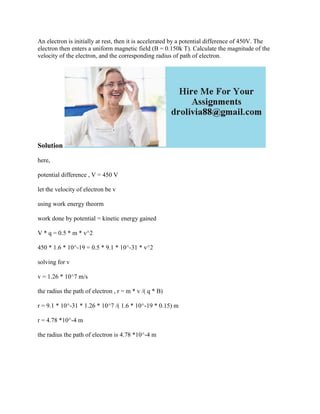Successfully reported this slideshow.

# An electron is initially at rest- then it is accelerated by a potentia.docx×

# An electron is initially at rest- then it is accelerated by a potentia.docx

An electron is initially at rest, then it is accelerated by a potential difference of 450V. The electron then enters a uniform magnetic field (B = 0.150k T). Calculate the magnitude of the velocity of the electron, and the corresponding radius of path of electron.
Solution
here,
potential difference , V = 450 V
let the velocity of electron be v
using work energy theorm
work done by potential = kinetic energy gained
V * q = 0.5 * m * v^2
450 * 1.6 * 10^-19 = 0.5 * 9.1 * 10^-31 * v^2
solving for v
v = 1.26 * 10^7 m/s
the radius the path of electron , r = m * v /( q * B)
r = 9.1 * 10^-31 * 1.26 * 10^7 /( 1.6 * 10^-19 * 0.15) m
r = 4.78 *10^-4 m
the radius the path of electron is 4.78 *10^-4 m
.

An electron is initially at rest, then it is accelerated by a potential difference of 450V. The electron then enters a uniform magnetic field (B = 0.150k T). Calculate the magnitude of the velocity of the electron, and the corresponding radius of path of electron.
Solution
here,
potential difference , V = 450 V
let the velocity of electron be v
using work energy theorm
work done by potential = kinetic energy gained
V * q = 0.5 * m * v^2
450 * 1.6 * 10^-19 = 0.5 * 9.1 * 10^-31 * v^2
solving for v
v = 1.26 * 10^7 m/s
the radius the path of electron , r = m * v /( q * B)
r = 9.1 * 10^-31 * 1.26 * 10^7 /( 1.6 * 10^-19 * 0.15) m
r = 4.78 *10^-4 m
the radius the path of electron is 4.78 *10^-4 m
.